# AP Physics 1: Vectors Multiple-Choice Practice Questions

### Test Information10 questions18 minutes

1. Two vectors, A and B, have the same magnitude, m, but vector A points north whereas vector B points east. What is the sum, A + B ?

2. If F1= -20ĵ, F2= -10î, and F3 = 5î + 10ĵ, what is the sum F1 + F2 + F3 ?

3. Both the x- and y-components of a vector are doubled. Which of the following describes what happens to the resulting vector?

4. If vectors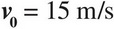north and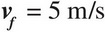south, what is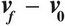?

5. The magnitude of vector A is 10. Which of the following could be the components of A ?

6. If the vector A = î - 2ĵ and the vector B = 4î - 5ĵ, what angle does A + B form with the x-axis?

7. An object travels along the vector d1 = 4m î + 5m ĵ and then along the vector d2 = 2m î - 3m ĵ. How far is the object from where it started?

8. If A =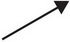and B =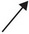, which of the following best represents the direction of A - B ?

9. The x-component of vector A is -42, and the angle it makes with the positive x-direction is 130°. What is the y-component of vector A ?

10. If two non-zero vectors are added together, and the resultant vector is zero, what must be true of the two vectors?